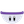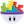#### Howdy, Stranger!

It looks like you're new here. If you want to get involved, click one of these buttons!

# Help me connect the dots with expressions

Posts: 70Memberedited August 2013
I want to get a better grasp on the expression editor. I've read about the expressions in the manual and looked at some examples.

I also found this tutorial by Darren from Deep Blue Apps which is SUPER helpful but I am having trouble seeing how this relates to the real world sin and cosine that I have been learning about lately. I only made took college algebra so I never had geo, trig or calc. But I have been teaching myself lately on youtube and working problems on paper. But thinking back to the expression editor I am having trouble connecting the dots.

First, starting simple with Pythagorean theorem:

1. I have seen a couple of people on these forums say use the Pythagorean theorem (A^2 + B^2 = C^2) as a basic example to teach students the expression editor as a basic starting point. I under stand how to use it to find area in real life. But I heard you can also use it to find the distance between two actors. How would you do that? And what is an example of an in game situation that I would want to do that?

I also assume the point that gamesalad would use to measure something like this is the very center of the actor unless an offset specifically entered into the expression editor right?

And to enter the offset you would add a negative or positive value to the x and y positions of the center pixel?

2. How would I start to enter the Pythagorean theorem into the expression editor? What expression would I use? I couldn't just type (A^2 + B^2 = C^2) into the editor could I? Or do I say something like constrain the x and y values of actor A and then again for B. Then in the expression editor would I use an exponent to the second power to the x and y positions? or is it as simple as saying Actor A^2 + Actor B^2 = Actor C^2? Im totally confused by this thought process.

Referencing the video by Darren from Deep Blue Apps I see how he is using sin and cosine to rotate one actor around another. While I get what he is doing to a small extent, I dont see the big picture and how it relates to real world trig expressions. I understand how tan = opp/adj, cos = adj/hyp, and sin = opp/hyp are used to find angles and measurements on triangles. And I can see how the degrees relate to rotating around the actor in the video and how they relate to sin etc. But do I use "tan = opp/adj, cos = adj/hyp, and sin = opp/hyp" as expressions in the expressions editor? Or is it simpler than that even?

In the video Darren has the X constrained with game.hereX + 150 * cos game.angle

What does that mean exactly? The 150 is the offset I was talking about above. But why multiple the offset by cos? Is that saying multiply 150 times adj/hye? This isn't a triangle so is it using the 4 right angles around the 0,0 coordinate of a graph? I assume GameSalad is the upper right portion of a graph since 0,0 is the lower left corner.

I think I am overly complicating things but maybe someone can explain this to me like a child then I will have an AHA moment... Part of me wonders how much of what I just typed is complete nonsense too. lol

• Posts: 2,824Member, Sous Chef, PROedited August 2013
None of what you typed is "complete nonsense". Your questions are exactly the right ones to be asking.

Unfortunately, you are asking for someone to give you a course in trig. I don't think anyone can help you understand the conceptual leap you need with just a few quick replies.

But we can help you with getting started. And we can show a couple of quick tricks. They might seem like magic incantations at first. But if you continue to ask the right questions you will have an "aha" moment at some point. (And perhaps at many points.)

First, GameSalad has a built in function that calculates distance for you. (So you don't need to remember exponents and such.) It is called "Magnitude" and is found in the second menu of the expression editor. For example, if you want to find the distance between the mouse and the actor you could put the following rule/behavior into an actor:

Rule (when mouse is down)
-- Change Attribute: distanceToMouse To: magnitude(game.Mouse.Position.X-self.Position.X,game.Mouse.Position.Y-self.Position.Y )

And that's it! Gamesalad will calculate the distance for you.

Hope that helps!
• Posts: 9,934Member, Sous Chef, Senior Sous-ChefWe do have ORBZ Trig 101 Demo available free at Gshelper

http://gshelper.com/shop/free-templates-and-tutorials/orbzample-trig-101/

Lots of cool stuff in there.

It's not my fault I never learned to take responsibility for anything.• Posts: 70MemberSorry for the late reply guys. Haven't been able too. Thank you for the replies. I just downloaded the trig tutorial from gshelper as well as the other 3 that popped up when I search for "trig". Maybe I am trying to understand the format in which gamesalad takes the expression from a normal trig expression. I am able to teach my self trig, that's not a problem. I guess I am trying to understand both as I learn trig. I see these expressions in the trig stuff I am learning. The basic find angle based on what you know about the hyp or opp side, or even as simple as the pyth theorem. But I guess what I dont understand is how to take one of the simple expression I see when I learn trig and translate it to game salad. If just this question alone is making it to where I am asking someone to teach me trig then I guess I just need to keep studying...
• Posts: 2,824Member, Sous Chef, PROHere is the standard distance formula worked out with GameSalad:
Constrain Attribute: self.myReallyLongDistanceFormula To: sqrt((pow(( game.Mouse.Position.X - self.Position.X ),2)+pow(( game.Mouse.Position.Y - self.Position.Y ),2)))

But to be honest, its been so long since I used it that I had to look it up on Google. Its much easier to use the magnitude function that is built in to GameSalad.
• London, UK.Posts: 12,822Memberedited September 2013

What does that mean exactly? The 150 is the offset I was talking about above. But why [multiply] the offset by cos?

@osakamitsu

Depending on the angle you've entered into the brackets - cos will return the cosine of a unit circle - a unit circle has radius of 1 so cosine's range is -1 to 1 - so you must multiply your offset by cosine (in reality you are multiplying cosine by your offset) to get any kind of useful value.

Hope than makes sense.
• Posts: 2,824Member, Sous Chef, PRO@osakamitsu -- You originally asked at least three questions. I was trying to answer questions one and two. While Socks is trying to answer question three.

You seem to have lost interest in your first two questions. So in order to avoid confusion I will back off of this discussion.
• Posts: 70Memberedited September 2013
No, I am sorry I haven't lost interest in any of it. Just been buy and haven't had time to make detailed replies. I was also just replying to what I thought was the immediate discussion.

I really appreciate all the help. I will dig into the gshelper stuff tomorrow night at work. It's weirdly when I have the most time for gamesalad stuff. I work a job where I can sit around and do nothing but gamesalad stuff. I'm very fortunate.

So taking at looking them in order since my last comment.

Regarding question 1 and 2:

RThurman:

Here is the standard distance formula worked out with GameSalad:
Constrain Attribute: self.myReallyLongDistanceFormula To: sqrt((pow(( game.Mouse.Position.X - self.Position.X ),2)+pow(( game.Mouse.Position.Y - self.Position.Y ),2)))

Ok so the distance formula is:

d= √(X₂-X1)^2+(Y₂-Y1)^2 (couldn't get the subscript to work for the 1's or the superscript on this forum)

Im trying to understand how what you said above relates to this. And how when i see sine and cosine expressions like this how do I do the same. Im also not sure what pow means in the above. To the power I assume? If so, why do you use that and not the exponent function? The way I see the above written is this:

d (the product) = √ pow??(x1 being the actors position - x2 the mouses x position) + pow(Y1 position of the mouse - the Y2 position of the actor)

I am sure it's easier to use magnitude but I am just trying to understand it before I take a short cut.

Edit: I would also like to ask about the x1/x2 and Y1/Y2 (numbers are subscript). So in the distance formula you have x1 and y1 as the actors positions and x2 and y2 as the mouse position. Should I assume that the self/whatever actor the expression is being applied to will always be x1/y1? Is there a reason you chose the mouse as the second x2/y2? Would it work the other way around? I understand the expression is measuring the distance between the actor and the mouse though. Backwards I guess would be the mouse and then the actor position, but would that just be weird that way?

What if you were to say make an infinite runner and wanted to measure the distance the actor made it through the level. How would you do this? I guess measure the magnitude of the x distance with how long the player has run for? Would you have to convert the time to the distance since you are probably spawning platforms to save memory, and wouldn't have the actual distance on the game scene? How would you use this to define a distance other than pixels, like meters?

Regarding question 3:

Socks:

What did I mean by offset? Probably bad terminology since I am a noob at this. I meant that the x and y value by default is the center of the actor, so adding the +150 would be adjust the center point. correct?

When you say a unit circe has a radius of 1 is that a unit that is specific to game salad?

Then the +150 I add then multiply by cosine would have what effect to the circle? The +150 would effect the radius right?

And yes all of this discussion is very much helping. Just trying to connect the math I have done on paper to practical stuff in gamesalad.

Edit:
And searching the forums I found another topic you were involved it with a lot of the stuff were talking about here.

I see that there are expression for rotating and actor clockwise and counter clockwise:

Clockwise using only 'sin':
X = AAA*sin( game.Time *BBB)+CCC
Y = AAA*sin( game.Time *BBB+90)+CCC

Counterclockwise using only 'sin':
X = AAA*sin( game.Time *BBB)+CCC
Y = AAA*sin( game.Time *BBB-90)+CCC